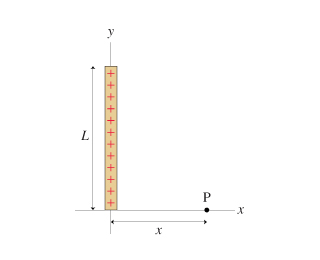# Problem: The figure (Figure 1) shows a thin rod of length L with total charge Q. Find an expression for the electric field E at distance X from the end of the rod. Give your answer in component form. Express your answer in terms of the variables Q, L, X, unit vectors i, j, and appropriate constants.

###### FREE Expert Solution

We consider a small element of length dl with a charge dq since the charge is uniformly distributed on the rod.

The electric field at point P due to charge element, dq is:

$\overline{)\stackrel{\mathbf{⇀}}{\mathbf{E}}{\mathbf{=}}\frac{\mathbf{d}\mathbf{q}}{\mathbf{4}\mathbf{\pi }{\mathbf{\epsilon }}_{\mathbf{0}}{\mathbf{r}}^{\mathbf{3}}}\stackrel{\mathbf{⇀}}{\mathbf{r}}}$

The charge element, dq is expressed as:

$\overline{){\mathbf{d}}{\mathbf{q}}{\mathbf{=}}\frac{\mathbf{Q}}{\mathbf{L}}{\mathbf{d}}{\mathbf{l}}}$

83% (121 ratings)###### Problem Details

The figure (Figure 1) shows a thin rod of length L with total charge Q. Find an expression for the electric field E at distance X from the end of the rod. Give your answer in component form. Express your answer in terms of the variables Q, L, X, unit vectors i, j, and appropriate constants.